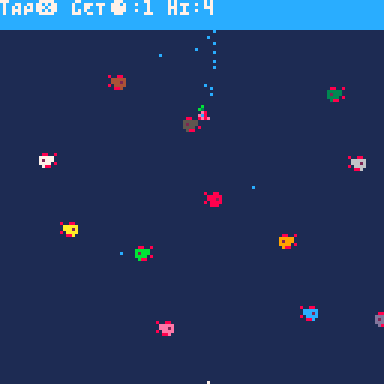### The Pico MermaidSingle button, pearl-fishing, 560 character PICO-8 game for Tweet Tweet Jam 5

9th Nov 2020

Use (X) to control the Pico Mermaid as she fetches pearls from the bottom of the sea back to the surface. Avoid the piranhas that will swim faster and faster as the mermaid retrieves more pearls.

This is my entry into Tweet Tweet Jam 5 and so the code fits into 560 characters (two tweets).

#### Features:

• Single-button controls (X)
• Animated and multi-colour pixel art sprites*
• air and water physics*
• Two particle systems*
• Difficulty ramp*
• Score effect*
• Death effect*
• start animation*
• current score and high score display*
• in-game instructions*

(* kinda)

Here's the code:

```P=pset::A::x=64y=0v=-9t=0w=127e=0d=.6s=0for z=0,29do
poke(z\6*x+z%6+1,'0x'..sub('e0e800800880ce0800444404e008002444043b0000444480b000004088',1+z*2,2+z*2))end::B::P(69,e<1and w or y,7)H=max(s,H)e=y>119and 1or e
?'tAP❎ gET●:'..s..' hI:'..H
v+=.5C=cls
if(y>8)v-=max(btnp(❎)and 2or.4,v-.6)
flip()C(1)rectfill(0,0,w,9,12)t=(t+d)%w
y=min(120,y+v)spr(0,x,y,1,1,t&8<1,e<1)for i=3,14do
d=-d
t=-t
h=40*i-t-4&w
pal(4,i)k=i*8k+=7*sin(h/w)if((h+4)\8==8and 4>abs(k-y-2))C(8)goto A
spr(1,h,k,1,1,d<0)P(rnd(8)+x,h*d%y+3)P(k*d/.7,h\d)end
if(y<9and e>0)e=0s+=1d*=-1.2C(7)
goto B```